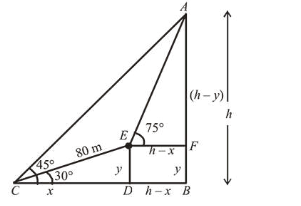# The angle of elevation of the top of a hill fromQuestion:

The angle of elevation of the top of a hill from a point on the horizontal plane passing through the foot of the hill is found to be $45^{\circ}$. After walking a distance of 80 meters towards the top, up a slope inclined at an angle of $30^{\circ}$ to the horizontal plane, the angle of elevation of the top of the hill becomes $75^{\circ}$. Then the height of the hill (in meters) is_____________.

Solution:

Let height $(A B)=h \mathrm{~m}, C D=x \mathrm{~m}$ and $E D=y \mathrm{~m}$In rt. $\Delta C D E$,

$\sin 30^{\circ}=\frac{y}{80} \Rightarrow y=40$

$\cos 30^{\circ}=\frac{x}{80} \Rightarrow x=40 \sqrt{3}$

Now, in $\triangle A E F$,

$\tan 75^{\circ}=\frac{h-y}{h-x}$

$\Rightarrow(2+\sqrt{3})=\frac{h-40}{h-40 \sqrt{3}}$

$\Rightarrow(2+\sqrt{3})(h-40 \sqrt{3})=h-40$

$\Rightarrow 2 h-80 \sqrt{3}+\sqrt{3} h-120=h-40$

$\Rightarrow h+\sqrt{3} h=80+80 \sqrt{3}$

$\Rightarrow(\sqrt{3}+1) h=80(\sqrt{3}+1)$

$\therefore h=80 \mathrm{~m}$